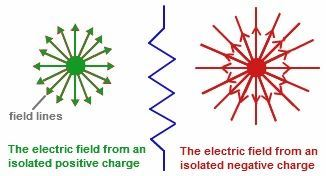# Electric Field Formula

Electric Field is the region produced by an electric charge around it whose influence is observed when another charge is brought in that region where the field exists.The force F experienced by electric charge q describes the Electric field lines.

## Formula of Electric Field

The Electric Field formula is expressed by

$$\begin{array}{l}E=\frac{F}{q}\end{array}$$

If q and Q are two charges separated by distance r, the Electric force is given by

$$\begin{array}{l}F = \frac{KqQ}{r^2}\end{array}$$
$$\begin{array}{l}E = \frac{KqQ}{r^2q}\end{array}$$

When we substitute the electric force formula in the electric field formula, we get Electric Field Formula which is given by,

If the voltage V is supplied across the given distance r, then the electric field formula is given as

$$\begin{array}{l}E = \frac{V}{r}\end{array}$$

The Electric field is measured in N/C.

### Solved Examples

Example 1

A force of 5 N is acting on the charge 6 μ C at any point. Determine the electric field intensity at that point.

Solution

Given

Force F = 5 N

Charge q = 6 μ C

Electric field formula is given by

E = F / q

= 5N / 6×10−6C

E = 8.33 × 105 N/C.